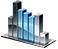# Object Math

Math object allows calculating values of common mathematic and trigonometric functions.

## Object methods:

Method nameReturn value
double Math.Abs(double val) Modulus of a `val` number, sent to the method as an argument.
double Math.Arccos(double val) The arccosine value of a number `val` where `val` must be within the range [-1;1]. The return value lies in the range from 0 to π radians.
double Math.Arcsin(double val) The arcsine value of a number `val` where `val` must be within the range [-1;1]. The return value lies in the range from -π/2 to π/2 radians.
double Math.Arctan(double val) The arctangent value of a number `val`. The return value lies in the range from -π/2 to π/2 radians.
double Math.Ceil(double val) The value that is the lowest integer which is more or equal to `val`.
double Math.Cos(double val) Cosine of an angle `val` that is specified in radians.
double Math.Exp(double val) The value of the number e raised to the power of `val`.
double Math.Floor(double val) The value that is the highest integer number which is less or equal to `val`.
double Math.Log(double val) The natural logarithm of the `val` number where `val` must be more than zero.
double Math.Max(double val1, double val2) The maximum value of `val1` or `val2`.
double Math.Min(double val1, double val2) The minimum value of `val1` or `val2`.
double Math.Mod(double val1, double val2) Remainder of the division `val1` by `val2`.
double Math.Pow(double base, double exp) The `base` value raised to the power of `exp`.
double Math.Rand() A pseudorandom value within the range from 0 to 32767.
double Math.Sin(double val) The sine of an angle `val`, specified in radians.
double Math.Sqrt(double val) The square root of `val` number where `val` must be more or equal to zero.
double Math.Srand(int seed) Sets the starting state for generating pseudorandom integer values.
double Math.Tan(double val) The tangent of an angle `val` specified in radians.